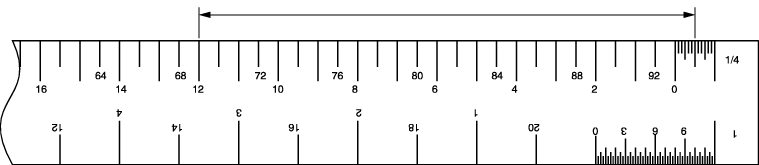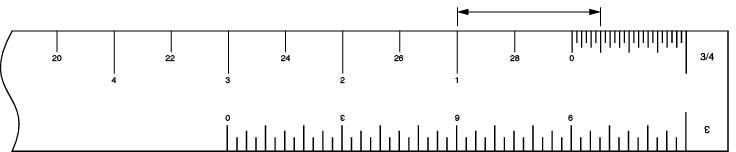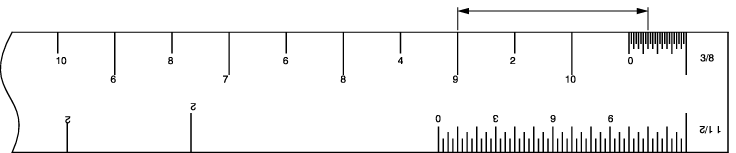# 3.E: Self Test 3

Self-Test 3

1. Scale rulers are available in both imperial and metric.
1. True
2. False
2. Which scale ruler would be most likely to have a ¼" to 1' scale on it?
1. Metric scale ruler
2. Architect’s scale ruler
3. Civil engineer’s scale ruler
4. Mechanical engineer’s scale ruler
3. How many scale ratios per edge do metric scale rulers have?
1. 1
2. 2
3. 3
4. 4
4. What is the best way to get exact dimensions from a drawing?
1. Measure using a tape measure.
2. Exact dimensions aren’t important.
3. Scale it with your combination square.
4. Use the dimension written between the dimension lines.
5. If a line measures 4½", what is the equivalent in 1/4"=1' scale?
1. 9"
2. 18'
3. 9'
4. 18"
6. If a line measures 63/8", what is the equivalent in 1/8" = 1' scale?
1. 27"
2. 48'
3. 4'3"
4. 51'
1. What is the measurement of the line below?1. 68'
2. 6'3"
3. 80'
4. 12'6"
2. What is the measurement of the line below?1. 1'3"
2. 1'6"
3. 27'3"
4. 27'6"
3. What is the measurement of the line below?1. 16'2"
2. 16'4"
3. 16'6"
4. 16'9"
1. What is the measurement of the line below?1. 3'4"
2. 3'7"
3. 9'4"
4. 13'7"
2. What is the measurement of the line below?1. 35 mm
2. 3.5 m
3. 35 m
4. 350 m
3. What is the measurement of the line below?1. 0.19 m
2. 1.9 m
3. 19 m
4. 190 m
1. What is the measurement of the piping run below in 1/2"=1' scale?1. 8'6"
2. 10'6"
3. 17'
4. 34'